# Right Δ

A right triangle has the length of one leg 7 cm and length of the hypotenuse 25 cm. Calculate the height of the triangle.

Result

vc =  6.7 cm

#### Solution:

$a = 7 \ cm \ \\ c = 25 \ cm \ \\ b = \sqrt{ c^2-a^2 } = 24 \ cm \ \\ \ \\ S = S \ \\ \dfrac{1}{2} c v_c = \dfrac{1}{2} a b \ \\ c v_c = a b \ \\ c v_c = a v_c = \dfrac{a}{c} b = \dfrac{ 7}{ 25} \cdot 24 = 6.7 \ \text { cm }$

Try calculation via our triangle calculator.

Leave us a comment of this math problem and its solution (i.e. if it is still somewhat unclear...):

Showing 1 comment:Math student
Maths

1 year ago  1 Like#### Following knowledge from mathematics are needed to solve this word math problem:

Do you have a linear equation or system of equations and looking for its solution? Or do you have quadratic equation? Pythagorean theorem is the base for the right triangle calculator. Do you want to convert length units? See also our trigonometric triangle calculator.

## Next similar math problems:

1. Land boundaryThe land has the shape of a right triangle. The hypotenuse has a length of 30m. The circumference of the land is 72 meters. What is the length of the remaining sides of the land boundary?
2. A rectangle 2A rectangle has a diagonal length of 74cm. It’s sides are 5:3. Find its lengths.
3. Sides of right angled triangleOne leg is 1 m shorter than the hypotenuse, and the second leg is 2 m shorter than the hypotenuse. Find the lengths of all sides of the right-angled triangle.
4. Isosceles triangle 10In an isosceles triangle, the equal sides are 2/3 of the length of the base. Determine the measure of the base angles.
5. The trapeziumThe trapezium is formed by cutting the top of the right-angled isosceles triangle. The base of the trapezium is 10 cm and the top is 5 cm. Find the area of trapezium.
6. Frustum of a cone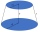A reservoir contains 28.54 m3 of water when completely full. The diameter of the upper base is 3.5 m while at the lower base is 2.5 m. Determine the height if the reservoir is in the form of a frustum of a right circular cone.
7. Parallels and one secant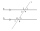There are two different parallel lines a, b and a line c that intersect the two parallel lines. Draw a circle that touches all lines at the same time.
8. FlowerbedWe enlarge the circular flower bed, so its radius increased by 3 m. The substrate consumption per enlarged flower bed was (at the same layer height as before magnification) nine times greater than before. Determine the original flowerbed radius.
9. Positional energyWhat velocity in km/h must a body weighing 60 kg have for its kinetic energy to be the same as its positional energy at the height 50 m?
10. Cylinder and its circumferenceIf the height of a cylinder is 4 times its circumference c, what is the volume of the cylinder in terms of its circumference, c?
11. An animal shelter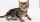An animal shelter spends $5.50 per day to care for each bird and$8.50 per day to care for each cat. Nicole noticed that the shelter spent \$283.00 caring for birds and cats on Wednesday. Nicole found a record showing that there were a total of 40 birds and
12. Square sideIf we enlarge the square side a = 5m, its area will increase by 10,25%. How many percent will the side of the square increase? How many percent will it increase the circumference of the square?
13. Heptagonal pyramidA hardwood for a column is in the form of a frustum of a regular heptagonal pyramid. The lower base edge is 18 cm and the upper base of 14 cm. The altitude is 30 cm. Determine the weight in kg if the density of the wood is 0.10 grams/cm3.
14. Blueberries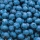5 children collect 4 liters of blueberries in 1.5 hours. a) How many minutes do 3 children take 2 liters of blueberries? b) How many liters of blueberries will be taken by 8 children in 3 hours?
15. Before yesterdayHe merchant adds a sale sign in his shop window to the showed pair of shoes in the morning: "Today by p% cheaper than yesterday. " After a while, however, he decided that the sign saying: "Today 62.5% cheaper than the day before yesterday". Determine the n
16. AM of three numbersThe number 2010 can be written as the sum of 3 consecutive natural numbers. Determine the arithmetic mean of these numbers.
17. Propeller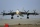The aircraft propeller rotates at an angular speed of 200 rad/s. A) What is the speed at the tip of the propeller if its distance from the axis of rotation is 1.5 m? B) What path does the aircraft travel during one revolution of the propeller at a speed